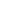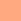The color coding means:: all participate: Doctoral students participate

Date Time Topic Speaker Room
2015 Apr 14 13:30-16:00 Qualitative Ratio and Weight Quantiles Daniel Krähmann TU Dresden INF E005
Forbidden permutation patterns and constraint satisfaction problems Tom Hanika
(TU Dresden)
2015 Apr 21 13:30-15:00 Structural and Epistemic Approaches to Probabilistic Argumentation Anthony Hunter
(University College London)
Universität Leipzig P 501
Felix-Klein-Hörsaal
2015 May 05 13:30-15:00 From PSL to Transition-based Generalized Büchi Automata Alexandre Duret-Lutz
(Epita)
TU Dresden INF E005
2015 May 12 13:30-16:00 On quantitative first-order logic George Rahonis
(Aristotle University of Thessaloniki)
Universität Leipzig P 501
Felix-Klein-Hörsaal
2015 May 19 13:30-15:00 Stochastic Semantics George Rahonis
(Aristotle University of Thessaloniki)
Universität Leipzig P 501
Felix-Klein-Hörsaal
2015 Jun 02 13:30-15:00 P ≠ P: Why Some Reasoning Problems are More Tractable Than Others Markus Krötzsch
(TU Dresden)
TU Dresden INF E005
2015 Jun 09 13:30-16:00 Do unambiguous automata suit Markov chains? David Müller TU Dresden INF E005
Open problems session all Ph.D. students
2015 Jun 16 09:00-18:00 Interviews   Universität Leipzig
Beratungsraum A 520
Neues Augusteum, 5. Stock
2015 Jun 30 13:30-15:00 Interactive Markov Chains (a short overview) Kirstin Peters
(TU Berlin/Dresden)
TU Dresden INF E005
2015 Jul 07 13:30-16:00 Logical Characterization of +ω-picture languages Parvaneh Babari Universität Leipzig P 501
Felix-Klein-Hörsaal
Deterministic omega-Automata for LTL: A safraless, compositional and mechanically verified construction
Cancelled due to illness
Salomon Sickert
(TU München)
2015 Jul 14 13:30-15:00 Decidability and expressiveness of synchronizing Markov Decision processes and synchronizing Probabilistic automata Mahsa Shirmohammadi
(University of Oxford)
Universität Leipzig P 501
Felix-Klein-Hörsaal

### Daniel Krähmann: Qualitative Ratio and Weight Quantiles

Using probabilistic model-checking techniques, quantiles and ratio objectives allow to reason about energy-utility trade-offs in Markov chain models. Whereas former approaches focused on quantiles and ratio objectives in isolation, we deal with a combination thereof. In particular, we show that qualitative ratio quantiles can be efficiently computed. That is, the exact computation of optimal thresholds, which are almost surely or with positive probability exceeded by the ratio between energy and utility, can be done in polynomial time. We also provide polynomial-time algorithms solving related decision problems on ratio and weight objectives.
This is joint a work with Christel Baier, Clemens Dubslaff, and Jana Schubert.

### Tom Hanika: Forbidden permutation patterns and constraint satisfaction problems

We investigate a new class of constraint satisfaction problems in the context of forbidden permutation patterns.
For a set of permutations P the set of permutations avoiding all elements of P is denoted by A(P). We define Forb-Perm-CSP(P) to be the computational problem of deciding whether two given binary relations on a finite set can be extended to linear orders coding a permutation in A(P).
Our goal is to classify the complexity of this problem for all finite sets of permutations P. We present polynomial-time algorithms for this problem in the case that P equals {231, 312}, {132, 213}, and {3142, 2413}. For these permutations, A(P) is known as the class of layered permutations, reverse layered permutations, and separable permutations, respectively.

### Anthony Hunter: Structural and Epistemic Approaches to Probabilistic Argumentation

Argumentation can be modelled at an abstract level using an argument graph (i.e. a directed graph where each node denotes an argument and each arc denotes an attack by one argument on another). Since argumentation involves uncertainty, it is potentially valuable to consider how this can quantified in argument graphs. In this talk, we will consider two probabilistic approaches for modeling uncertainty in argumentation. The first is the structural approach which involves a probability distribution over the subgraphs of the argument graph, and this can be used to represent the uncertainty over the structure of the graph. The second is the epistemic approach which involves a probability distribution over the subsets of the arguments, and this can be used to represent the uncertainty over which arguments are believed. The epistemic approach can be constrained to be consistent with Dung’s dialectical semantics, but it can also be used as a potential valuable alternative to Dung’s dialectical semantics. We will consider applications of probabilistic argumentation in handling enthymemes (arguments with incomplete premises) and in selecting moves in an argumentation dialogue.

### Alexandre Duret-Lutz: From PSL to Transition-based Generalized Büchi Automata

Couvreur [FM'99] has introduced a simple yet efficient algorithm to translate LTL formulas into Generalized Büchi Automata with Transition-based acceptance (TGBA), based on a tableau construction. These TGBA can then be used directly for model checking.

We show how to extend this algorithm to create TGBA from formulas expressed in the linear fragment of PSL, which basically are LTL formulas over regular expressions. Translating PSL formulas is typically done using alternating-automata (not 1-weak, as in the case of LTL). Here we mix some ideas of the Miyano-Hayashi construction for alternation removal with the tableau construction of Couvreur, in order to avoid constructing an alternating-automaton.

### George Rahonis: On quantitative first-order logic

We extend the well-known expressive equivalence of first-order logic, LTL, star free languages and counter-free Büchi automata in two quantitative setups. First, over the max-plus semiring with discounting, and second over complete, idempotent and zero-divisor free semirings. We state several open problems for future research.

### George Rahonis: Stochastic Semantics

We investigate stochastic systems of equations of tree series and consider their least [IO]- and OI-solutions in arbitrary substochastic algebras. We prove that it is decidable whether the components of these solutions are stochastic functions or not. For the class of stochastically OI-equational tree series, i.e., the components of the least OI-solutions of stochastic systems in the term algebra, we state a Kleene type theorem. We present two applications of our theory, namely the consistency problem for stochastic tree automata is decidable, and a Kleene theorem for the class of word series generated by stochastic context-free grammars.

### Markus Krötzsch: P ≠ P: Why Some Reasoning Problems are More Tractable Than Others

Knowledge representation and reasoning leads to a wide range of computational problems, and it is of great interest to understand the difficulty of these problems. Today this question is mainly studied using computational complexity theory and algorithmic complexity analysis. For example, entailment in propositional Horn logic is P-complete and a specific algorithm is known that runs in linear time. Unfortunately, tight algorithmic complexity bounds are rare and often based on impractical algorithms (e.g., O(n^2.373) for transitive closure by matrix multiplication), whereas computational complexity results abound but are very coarse-grained (e.g., many P-complete problems cannot be solved in linear time).

In this talk, we therefore advocate another approach to quantifying the difficulty of a computation: we reformulate computational problems as query answering problems, and then ask how powerful a query language is needed to solve these problems. This reduces reasoning problems to a computational model – query answering – that is supported by many efficient implementations. It is of immediate practical interest to know if a problem can be reduced to query answering in an existing database system. On the theoretical side, it allows us to distinguish problems in a more-fine grained manner than computational complexity without being specific to a particular algorithm. We provide several examples of this approach and discuss its merits and limitations.

### Kirstin Peters: Interactive Markov Chains (a short overview)

The talk will provide a short introduction into interactive Markov Chains. Interactive Markov Chains are an extension of labelled transition systems with exponentially delayed transitions. They are closed under parallel composition and hiding. Interactive Markov Chains are a natural semantic model for stochastic process algebras.

### Parvaneh Babari: Logical Characterization of +ω-picture languages

Languages of finite and infinite two-dimensional words (pictures and ωω-pictures, respectively) have been considered by several research groups, and several formal models to recognize or generate finite or infinite two-dimensional objects have been proposed in literature. Here, we define a new class of two-dimensional languages and we call them +ω-picture languages. These are pictures with finite number of rows but infinite number of columns. We have already characterized this family by three different devices, namely, a kind of automata device called Büchi two-dimensional on-line tessellation automata, tiling systems with a Büchi accepting conditions and also domino systems, and we obtained an equivalence theorem for +ω-picture languages describing recognizable languages in terms of our automata model, sets of tiles and sets of dominoes. In this paper, we solve the open problem regarding logical characterization of +ω-picture languages. We define an existential monadic second-order logic which is equipped with an existential quantifier that is applied to second-order variables for infinite sets and we prove that the class of +ω-picture languages is Büchi tiling systems recognizable if and only if it is definable in terms of our existential monadic second-order logic.

### Salomon Sickert: Deterministic omega-Automata for LTL: A safraless, compositional and mechanically verified construction

Recently a new method directly translating linear temporal logic (LTL) formulas to deterministic (generalized) Rabin automata was proposed by Javier Esparza and Jan Křetínsḱy. Compared to the existing approaches of constructing a non-deterministic Buechi-automaton in the first step and then applying a determinization procedure (e.g. some variant of Safra's construction) in a second step, this new approach preservers a relation between the formula and the states of the resulting automaton. While the old approach produced a monolithic structure, the new method is compositional. Furthermore it was shown in experiments that the resulting automata were much smaller then the automata generated by existing approaches. In order to guarantee the correctness of the construction a full verification of it was done using Isabelle/HOL.

In my talk I will introduce the basic concepts and essential ideas of the construction.

### Mahsa Shirmohammadi: Decidability and expressiveness of synchronizing Markov Decision processes and synchronizing Probabilistic automata

Markov decision processes (MDPs) are finite-state probabilistic systems with both strategic and random choices, hence well-established to model the interactions between a controller and its randomly responding environment. An MDP is viewed as a 1/2-player stochastic game played in rounds when the controller chooses an action, and the environment chooses a successor according to a fixed probability distribution.

Recently, MDPs have been viewed as generators of sequences of probability distributions over states, called the distribution-outcome. We introduce synchronizing conditions defined on distribution-outcomes, which intuitively requires that some (group of) state(s) tend to accumulate all the probability mass. We consider the following modes of synchronization: the always mode where all distributions of the distribution-outcome must be synchronized, the eventually mode where some distributions must be synchronized, the weakly mode where infinitely many distributions must be synchronized, and the strongly mode where all but finitely many distributions must be synchronized.

For each synchronizing mode, we study three winning modes: sure, almost-sure and limit-sure. In this talk, we present the various synchronization modes and winning modes, as well as an overview of the key properties for their analysis.

We also give hints on synchronization in probabilistic automata (PAs), that are kind of MDPs where controllers are restricted to use only word-strategies; i.e. no ability to observe the history of the system execution, but the number of choices made so far.